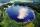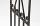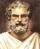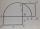# Clock

How many times a day hands on a clock overlap?

Result

n =  22

#### Solution:6m -0 = m/2; m = 2/11*0 = 0 min ==> 0:00:00
6m -360 = m/2; m = 2/11*360 = 65.45 min ==> 1:05:27
6m -720 = m/2; m = 2/11*720 = 130.91 min ==> 2:10:54
6m -1080 = m/2; m = 2/11*1080 = 196.36 min ==> 3:16:21
6m -1440 = m/2; m = 2/11*1440 = 261.82 min ==> 4:21:49
6m -1800 = m/2; m = 2/11*1800 = 327.27 min ==> 5:27:16
6m -2160 = m/2; m = 2/11*2160 = 392.73 min ==> 6:32:43
6m -2520 = m/2; m = 2/11*2520 = 458.18 min ==> 7:38:10
6m -2880 = m/2; m = 2/11*2880 = 523.64 min ==> 8:43:38
6m -3240 = m/2; m = 2/11*3240 = 589.09 min ==> 9:49:05
6m -3600 = m/2; m = 2/11*3600 = 654.55 min ==> 10:54:32
6m -3960 = m/2; m = 2/11*3960 = 720 min ==> 12:00:00
6m -4320 = m/2; m = 2/11*4320 = 785.45 min ==> 13:05:27
6m -4680 = m/2; m = 2/11*4680 = 850.91 min ==> 14:10:54
6m -5040 = m/2; m = 2/11*5040 = 916.36 min ==> 15:16:21
6m -5400 = m/2; m = 2/11*5400 = 981.82 min ==> 16:21:49
6m -5760 = m/2; m = 2/11*5760 = 1047.27 min ==> 17:27:16
6m -6120 = m/2; m = 2/11*6120 = 1112.73 min ==> 18:32:43
6m -6480 = m/2; m = 2/11*6480 = 1178.18 min ==> 19:38:10
6m -6840 = m/2; m = 2/11*6840 = 1243.64 min ==> 20:43:38
6m -7200 = m/2; m = 2/11*7200 = 1309.09 min ==> 21:49:05
6m -7560 = m/2; m = 2/11*7560 = 1374.55 min ==> 22:54:32
6m -7920 = m/2; m = 2/11*7920 = 1440 min ==> 24:00:00 !!! h>23

6m -8280 = m/2; m = 2/11*8280 = 1505.45 min ==> 25:05:27 !!! h>23

n=22

Leave us a comment of example and its solution (i.e. if it is still somewhat unclear...):

Showing 1 comment:Bo Shag
I dislike these questions, but they are very good for the brain.#### To solve this verbal math problem are needed these knowledge from mathematics:

Do you solve Diofant problems and looking for a calculator of Diofant integer equations? Do you have a linear equation or system of equations and looking for its solution? Or do you have quadratic equation? Do you want to convert length units? Try conversion angle units angle degrees, minutes, seconds, radians, grads.

## Next similar examples:

1. RPMAn electric motor makes 3,000 revolutions per minutes. How many degrees does it rotate in one second?
2. Tangents constructCircle is given k (S; 2.5 cm) and an outer line p. Construct a tangent t of the circle that has with a line p angle 60°. How many solutions has the task?
3. The bridgeAcross the circle lakepasses through its center bridge over the lake. At three different locations on the lake shore are three fishermen A, B, C. Which of fishermen see the bridge under the largest angle?
4. Steel tubeThe steel tube has an inner diameter of 4 cm and an outer diameter of 4.8 cm. The density of the steel is 7800 kg/m3. Calculate its length if it weighs 15 kg.
5. The chordSide of the triangle inscribed in a circle is a chord passing through circle center. What size are the internal angles of a triangle, if one of them is 40°?
6. Circumferential angleVertices of the triangle ΔABC lies on circle and divided it into arcs in the ratio 2:2:9. Determine the size of the angles of the triangle ΔABC.
7. Complete constructionConstruct triangle ABC if hypotenuse c = 7 cm and angle ABC = 30 degrees. / Use Thales' theorem - circle /. Measure and write down the length of legs.
8. Inscribed triangleTo a circle is inscribed triangle so that the it's vertexes divide circle into 3 arcs. The length of the arcs are in the ratio 2:3:7. Determine the interior angles of a triangle.
9. Hypotenuse - RTA triangle has a hypotenuse of 55 and an altitude to the hypotenuse of 33. What is the area of the triangle?
10. RectangleIn rectangle with sides, 6 and 3 mark the diagonal. What is the probability that a randomly selected point within the rectangle is closer to the diagonal than to any side of the rectangle?
11. OK circleCalculate the radius (circumradius) of the circle described right triangle with hypotenuse long 33 and one cathetus long 17.
12. Right triangleDraw a right triangle ABC if |AB| = 5 cm |BC| = 3 cm, |AC| = 4 cm. Draw Thales circle above the hypotenuse of the triangle ABC.
13. SemicircleIn the semicircle with center S and the diameter AB is constructed equilateral triangle SBC. What is the magnitude of the angle ∠SAC?
14. Circle tangentIt is given to a circle with the center S and radius 3.5 cm. Distance from the center to line p is 6 cm. Construct a circle tangent n which is perpendicular to the line p.
15. Circle describedThe radius of the circle described to the right triangle with 6 cm long leg is 5 cm. Calculate the circumference of this triangle.
16. Same areaThere is a given triangle. Construct a square of the same area.
17. Touch x-axisFind the equations of circles that pass through points A (-2; 4) and B (0; 2) and touch the x-axis.